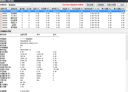1、哪句语句是画那条粗粗红色0线的？庄拉主升浪:20*(CROSS(庄拉线,黑马线) AND 黑马线>0 AND 量价线>0),LINETHICK3,COLOR0000FF;
2、为什么除了黑马线、量价线、量价先行画出线，且名称后会显示数值，而其他名称既没有不划线函数，也没画出线，又不显示数值？

3、小图标只看见10号图标，其他1、2、9号图标都不见？这些图标任何行情不显示，这些语句不是多余的吗？MX000:=(0.36094 ...NOTEXT:IF(REFX(MA(C,20)=REF(MA(C,20),1),1) OR MA(C,20)=REF(MA(C,20),1),M20,DRAWNULL),LINETHICK2,COLORRED;&quot;

300022吉峰科技，在2月9-10日两天，2月22-25日四天MA20均线【1】
M20:MA(C,20);
NOTEXT:IF(REFX(MA(C,20)=REF(MA(C,20),1),1) OR MA(C,20)=REF(MA(C,20),1),M20,DRAWNULL),LINETHICK2,COLORRED;
【2】
AB:=IF(总买!=DRAWNULL,1,0.97),NODRAW;
【3】JL:=MA(MB4,6);{均量}
{操作提示}

DRAWTEXT_FIX(CROSS(MB3>JL,JL)OR MB3<JL AND MB3>=JL AND ISLASTBAR,0.95,0.72,0,'买入'),COLOR2B71F2;
DRAWTEXT_FIX(MB7>盈利筹码 AND ISLASTBAR,0.974,0.15,0,'回调'),COLORWHITE;
DRAWTEXT_FIX(套牢筹码>JL AND ISLASTBAR,0.955,0.99,0,'买入'),COLORYELLOW;
DRAWTEXT_FIX(盈利筹码>JL AND 盈利↑ AND ISLASTBAR,0.903,0,0,'盈利趋多'),COLORLIRED;
DRAWTEXT_FIX(盈利筹码>JL AND 盈利↓ AND ISLASTBAR,0.903,0,0,'盈利趋少'),COLORYELLOW;
DRAWTEXT_FIX(盈利筹码>JL AND ISLASTBAR,0.955,0,0,'持股'),COLOR2B71F2;
DRAWTEXT_FIX(套牢筹码<JL AND 套牢↓ AND ISLASTBAR,0.955,0.99,0,'持币'),COLOR1CBC1C;
DRAWTEXT_FIX(套牢筹码<JL AND 套牢↑ AND ISLASTBAR,0.903,0.99,0,'趋势向好'),COLORLIRED;
DRAWTEXT_FIX(套牢筹码<JL AND 套牢↓ AND ISLASTBAR,0.903,0.99,0,'套牢趋多'),COLORWHITE;
DRAWTEXT_FIX(套牢筹码<JL AND 套牢↑ AND ISLASTBAR,0.85,0.99,0,'套牢趋少'),COLOR8DEBF8;
DRAWTEXT_FIX(套牢筹码>MB7 AND ISLASTBAR,0.974,0.99,0,'介入'),COLORYELLOW;BOLL:MA(CLOSE,20);
UB:BOLL+2*STD(CLOSE,20);
LB:BOLL-2*STD(CLOSE,20);

IF(CODELIKE('688') OR (CODELIKE('300') AND DATE>=1200824),C=ZTPRICE(REF(C,1),0.2),
C=ZTPRICE(REF(C,1),0.1)));

DRAWNUMBER(C>=O AND MOD(SUM(C>=O,白色高天数+1),2)=0 AND SUM(SUM(H<HHV(H,白色高天数),3)=3,白色高天数)=0,
H*1.04,SUM(C>=O,白色高天数+1)),COLORWHITE;
DT:=BARSLAST(L>LB AND REF(L<LB,1));
DRAWNUMBER(C>=O AND MOD(SUM(C>=O,DT+1),2)=1 AND SUM(H<UB AND REF(H>UB,1),DT+1)=0,L*0.97,SUM(C>=O,DT+1)),COLORGREEN;
GT:=BARSLAST(H<UB AND REF(H>UB,1));
DRAWNUMBER(IF(SUM(H<UB AND REF(H>UB,1),DT+1)=0 OR SUM(DOWNNDAY(H,3),白色高天数)=0,0,C>=O AND MOD(SUM(C>=O,GT+1),2)=0 AND SUM(C>=O AND L<LB,GT)=0),H*1.04,SUM(C>=O,GT+1)),COLORYELLOW;BETWEEN(M,30,60);BOLL:=MA(CLOSE,20);L-BOLL<=0.01  后次L>=前次L